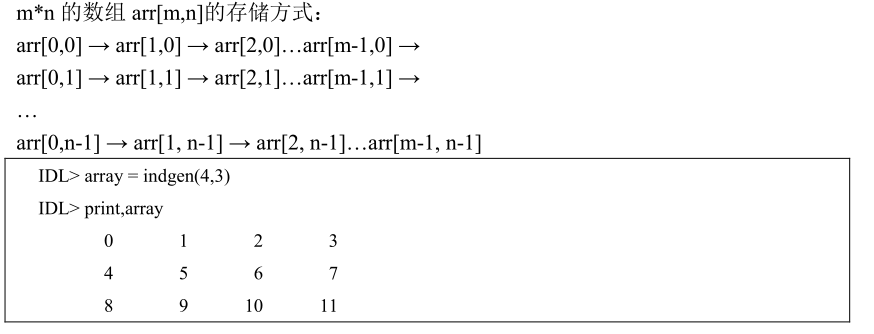• 今天又把numpy where函数的用法忘记了。赶紧上网搜了下，写的都不太好。最后在知乎上看到了很好的答案，一看就明白了where函数的用法，链接如下 where 函数用法...
今天又把numpy where函数的用法忘记了。赶紧上网搜了下，写的都不太好。最后在知乎上看到了很好的答案，一看就明白了where函数的用法，链接如下
where 函数用法
展开全文numpy python
• where函数介绍 函数WHERE()能返回数组中满足指定条件的元素下标 调用格式： Result=Where(数组表达式[,count][,Complement=变量1][,/L64][,NCOMPLEMENT=变量2]) 其中，关键字count返回符合指定条件的元素个数；变量1...
where函数介绍
函数WHERE()能返回数组中满足指定条件的元素下标 调用格式： Result=Where(数组表达式[,count][,Complement=变量1][,/L64][,NCOMPLEMENT=变量2]) 其中，关键字count返回符合指定条件的元素个数；变量1为不满足条件的数组元素下标；变量2为不满足条件的数组元素个数。 注意：当没有符合条件的元素时，返回值为-1。
where函数举例
a=[[1,2],[3,4]]
index = where(a ge 2,count,Complement=d,NCOMPLEMENT=opp_count)
print,index,count,d,opp_count
% Compiled module: FUNCTION_TEST.
1           2           3
3
0
1

index为数组a中大于等于2的元素的一维下标 count为满足数组a中大于等于2的元素数量 d则为不满足数组a中大于等于2的元素下标 opp_count为数组a中不满足大于等于2的元素个数。
关于IDL数组的下标可参考以下代码进行理解。 IDL中的矢量和数组均是以先行后列顺序存储的(IDL最初是设计用来处理行扫描的卫星数据)，下标值的起始值是 0。展开全文• 首先我们看一下Pytorch中torch.where函数是怎样定义的： @overload def where(condition: Tensor) -> Union[Tuple[Tensor, ...], List[Tensor]]: ... torch.where函数的功能如下： torch.where(condition, x...
首先我们看一下Pytorch中torch.where函数是怎样定义的：
@overload
def where(condition: Tensor) -> Union[Tuple[Tensor, ...], List[Tensor]]: ...
torch.where函数的功能如下：
torch.where(condition, x, y):
condition：判断条件
x：若满足条件，则取x中元素
y：若不满足条件，则取y中元素
以具体实例看一下torch.where函数的效果：
import torch

# 条件
condition = torch.rand(3, 2)
print(condition)
# 满足条件则取x中对应元素
x = torch.ones(3, 2)
print(x)
# 不满足条件则取y中对应元素
y = torch.zeros(3, 2)
print(y)
# 条件判断后的结果
result = torch.where(condition > 0.5, x, y)
print(result)
结果如下：
tensor([[0.3224, 0.5789],
[0.8341, 0.1673],
[0.1668, 0.4933]])
tensor([[1., 1.],
[1., 1.],
[1., 1.]])
tensor([[0., 0.],
[0., 0.],
[0., 0.]])
tensor([[0., 1.],
[1., 0.],
[0., 0.]])
可以看到torch.where函数会对condition中的元素逐一进行判断，根据判断的结果选取x或y中的值，所以要求x和y应该与condition形状相同。
展开全文python
• 官方解释是有些难以理解，我将个人理解分享如下： numpy.where的格式： numpy.where(condition,x,y) condition：类数组对象，...numpy.where分两种方式使用。 第一种：numpy.where(condition,x,y).condition为真...
官方解释是有些难以理解，我将个人理解分享如下：
numpy.where的格式：
numpy.where(condition,x,y)

condition：类数组对象，布尔逻辑（即True或False）。
x,y：类数组对象，optional(这个不太明白，不过不影响使用)
numpy.where分两种方式使用。
第一种：numpy.where(condition,x,y).condition为真，输出x;假，则输出y.
第二种：numpy.where(condition,x,y).condition为真， 返回conditon.nonzero().(返回condition（类数组对象）非零元素的坐标)
举例： 第一种
np.where([[True, False], [True, True]],
[[1, 2], [3, 4]],
[[9, 8], [7, 6]])

结果：
array([[1, 8],
[3, 4]])

这是官方的例子，刚开始不明白意思，但这样看就一目了然：
np.where([[True, False], [True, True]],
[[1   , 2    ], [3   , 4   ]],
[[9   , 8    ], [7   , 6   ]])

结果：
array([[1,8],[3,4]])

第一列分别对应conditon,x,y,以此类推第二列第三列均如此。 第一列,conditon为True,为逻辑真值，则输出x=1 第二列,False,输出y =8
第二种：
dataSet=array
( [[1,0,0,0],
[0,1,0,0],
[0,1,0,0],
[0,0,0,1]])
np.where(dataSet)

结果：
(array([0, 1, 2, 3], dtype=int64), array([0, 1, 1, 3], dtype=int64))

输出了非零元素的所在行，所在列位置。 ————————————————
展开全文• ## pandas where函数用法

万次阅读 2018-09-22 23:04:42
Series.where(cond, other=nan, inplace=False, axis=None, level=None, errors=‘raise’, try_cast=False, raise_on_error=None) 如果 cond 为真，保持原来的值，否则替换为other， inplace为真标识在原数据上操作...python pandas
• 本人最近对python的where函数产生了一些疑问，因为它和IDL中的where函数不太一样，本文主要就两种语言的where函数进行简单的比较。 1 . Python 的where函数在多条件下的判断 import numpy as np #首先创建两个非常...python
• WHERE简介 按照指定条件查找数组元素，并返回数组中满足条件的元素的下标。 语法： result=where(array_expression [,count] [,complement=variable],[,ncomplement=variable] ) array_expression ：数组表达式 ...
• np.where函数最常见的用法是： np.where(condition)，只有条件，函数返回满足条件元素的坐标（索引）.需要注意的是，这里的索引是以tuple的形式给出，因此原数组有多少维，输出的tuple就包含几个数组，分别对应符合...
• 关于模块pandas.core.component....中的函数where的帮助。 where(self, cond, other=nan, inplace=False, axis=None, level=None, errors='' raise', try_cast=False) 替换条件为False的值。 参数 con...
• 函数WHERE()能返回数组中满足指定条件的元素下标 调用格式： Result=Where(数组表达式[,count][,Complement=变量1][,/L64][,NCOMPLEMENT=变量2]) 其中，关键字count返回符合指定条件的元素个数；变量1为不满足...
• SQL中where语句能聚合函数吗?聚合函数应该在having子句中。 聚合函数如果想where中的话，应该只能子查询来实现(在子查询中进行聚合函数计算，然后在主查询中进行where判断)。为什么聚集函数不能出现在...
• where使用decode函数达到要求。 select * from zjk_pos_supplier_order where ((decode(:code, NULL, 1, 0) = 1) or code = :code) and order_date >= :order_date;  以上语句如果:code变量赋值为null...
• 但只使用where或者having时，作用一样（不能（慎使用聚合函数）。 where不能使用聚合函数 因为聚合函数是从确定的结果集中整列数据进行计算的 ，而where子句则是对数据行进行过滤的。所以相矛盾不能使用。 ...mysql sql 数据库
• 本篇文章主要介绍了详解Python中where()函数的用法，小编觉得挺不错的，现在分享给大家，也给大家做个参考。一起跟随小编过来看看吧
• 1.问题select department_id,avg...where avg(salary)>6000 group by department_id --having avg(salary)>6000 2.原因。 sql语句的执行顺序为  from子句 where 子句 group by 子句 having 子句 order byhaving
• ## tf.where()函数

万次阅读 多人点赞 2017-11-30 11:04:20
本篇博客翻译了tensorflow官网对tf.where()使用的API，并且我做了相应的演示python tensorflow
• Numpy的高级函数where和extract函数 照例我们先导入numpy库 #导入numpy import numpy as np 首先我们先要定义一个用于操作的numpy数组（numpy中的数组都是ndarray）： x=np.array([[1,2,3],[4,5,6],[7,8,9]]) ...python numpy AI人工智能 机器学习
• ## TensorFlow函数：tf.where

万次阅读 多人点赞 2018-01-12 21:49:13
前言 ...经过百度查找，发现有人使用tf.where来实现这个功能，但看官方文档看来好久（下午+晚上）才看明白这个函数是如何使用的，特在此记录下。 之后会专门写一篇有关Focal Loss的Keras实现的博客Keras
• 一、where函数 ** torch.where(condition,x,y) out = x,if condition is 1 = y ,if condition is 0 In : cond = torch.rand(2,2) In : cond Out: tensor([[0.1326, 0.4126], [0.7093, 0.5339]])...
• 当存在合计函数时，条件where无法执行 合计函数（Aggregate functions） Aggregate 函数的操作面向一系列的值，并返回一个单一的值。 注释：如果在 SELECT 语句的项目列表中的众多其它表达式中使用 ...
• 使用聚合函数count出一个数值作为查询结果的一部分，同时需要在where条件中利用count函数的结果做进一步筛选，需要用到having语句，如果添加到where中会报错 同时group by 和having 顺序不能调换 ...
• 今天小编就为大家分享一篇关于numpy.where()函数 返回值的解释，具有很好的参考价值，希望对大家有所帮助。一起跟随小编过来看看吧
• where子句 例： -- 查询销售人员或办事人员并且工资大于 800 select * from emp WHERE (job='CLERK' OR job='SALESMAN') and sal >800 -- 查询奖金介于1500-3000 select * from emp where sal between ...数据库 select web应用 web开发
• ## torch.where()函数解读

万次阅读 多人点赞 2019-10-12 16:48:27
torch.where()函数的作用是按照一定的规则合并两个tensor类型。 代码示例 >>> import torch >>> a=torch.randn(3,5) >>> b=torch.ones(3,5) >>> a tensor([[-0.0310, 1.5895, ...
• 1.问题描述 select deptno ,avg(sal) ... 在where 句中使用聚合函数count(*),报出错误：ORA-00934: group function is not allowed here 那是为什么呢？ 2.问题解决： 大致解释如下，sql语句的执行过程是：from-->where
• data.where(cond, other=nan, inplace=False, axis=None, level=None, errors=’raise’, try_cast=False, raise_on_error=None) Docstring: Return an object of same shape as self and whose corr...
• tf.where()函数的语法格式如下： import tensorflow as tf tf.where( condition, x=None, y=None, name=None ) 作用：该函数的作用是根据condition,返回相对应的x或y,返回值是一个tf.bool类型的Tensor。 例1： ...tensorflow 深度学习
• ## where()函数的用法

千次阅读 2019-09-29 19:20:19
#np.where()有两种用法： #1.np.where(condition, x, y) #满足条件(condition)，输出x，不满足输出y。 aa = np.arange(10) np.where(aa,1,-1) np.where(aa > 5,1,-1) np.where([[True,False], [True,True]],[[1,2...
• CASE WHEN 其实相当于产生了一个列，最后WHERE 再按那个列来限定 SELECT * AS A FROM TB WHERE CASE WHEN 1=1 THEN 1 ELSE 0 END =1 等价于 SELECT * FROM （SELECT * ，CASE WHEN 1=1 THEN 1 ELSE 0 END AS A ......